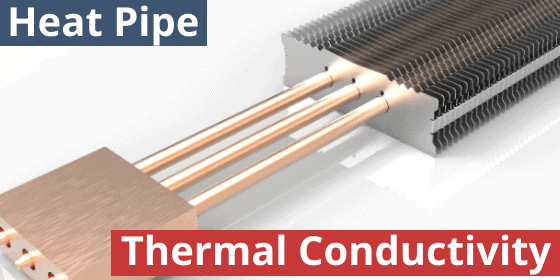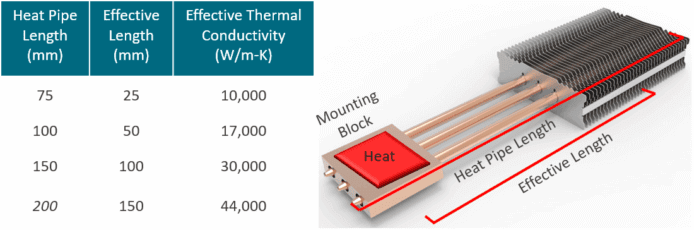# Heat Pipe Thermal ConductivityKnowing heat pipe thermal conductivity is important when performing Excel or CFD modeling of two-phase devices integrated into a heat sink assembly. In theory, heat pipe thermal conductivity can range from 4,000 to 100,000 W/m-K. In reality, the range for electronics cooling applications is more like 1,500 to 50,000 W/m-K. This is still an enormous improvement over the thermal conductivity of solid copper (390 W/m-K) or solid aluminum (200 W/m-K). This difference makes heat pipes an indispensable component for many of today’s high-performance heat sinks. Engineers need to confirm the thermal conductivity for each application because heat pipe thermal conductivity, unlike solid metals, varies with length (holding constant heat source power & size, and heat sink (evaporator) length.Figure 1: Heat Pipe Effective Thermal Conductivity as Function of Length

Figure 1 illustrates the effect of length on heat pipe thermal conductivity.  In this example, three heat pipes are used to transport heat from a 75 W power source.  While thermal conductivity of 10,000 W/m.K is achieved at just under 100 mm heat pipe length, a 200 mm length has less than one-third the typically published maximum thermal conductivity of 100,000 W/m.K.  As observed in the calculation for effective thermal conductivity in equation (1), the heat pipe effective length is a function of adiabatic, evaporator and condenser lengths:

Keff = Q Leff /(A ΔT)                                                       (1)

where:

Keff = Effective thermal conductivity [W/m.K]

Q = Power transported [W]

Leff = Effective length =  (Levaporator + Lcondenser)/2 + Ladiabatic  [m]

A = Cross-sectional area [m2]

ΔT = Temperature difference between evaporator and condenser sections [°C]

You can calculate heat pipe effective thermal conductivity using our online Heat Pipe Calculator. To find vapor chamber thermal conductivity use our online Heat Sink Calculator.

## Differences in Solid Metal vs Heat Pipe Thermal Conductivity

The thermal conductivity of a solid metal stays constant because it’s made up of all the same stuff, copper for instance. Consequently, each copper molecule has to pass heat to the next copper molecule. Sort of like an old-time bucket brigade. Copper thickness, length, or applied heat flux make no difference.

Heat pipe thermal conductivity, by contrast, has multiple stages of heat transfer. While it’s true that heat first needs to travel through the outer solid copper wall of the heat pipe, the heat transfer process gets accelerated during the next stage: liquid evaporation. During this stage, the working fluid, water in most cases, turns to vapor when heat is applied. And because the thermal resistance of vapor traveling down the heat pipe is so minimal it boosts thermal conductance. Moreover, the longer a distance the vapor travels (a longer heat pipe) the more the effective thermal conductivity of a heat pipe increases.

## Differences in Thermal Conductivity by Heat Pipe Diameter

Holding all other variables constant, heat pipe thermal conductivity changes with diameter, but not in the direction one might expect. Small diameter heat pipes, while having a lower Qmax, have a higher effective thermal conductivity than larger diameter pipes. This is because effective thermal conductivity goes down by the ratio of the cross-sectional area. Larger diameter heat pipes have larger cross-sections. This is also the same reason a vapor chamber for a particular application will have a lower thermal conductivity than the equivalent heat pipe(s) solution.

Refer to these two articles for information on two-phase designs: Heat Pipe Design Guide and Vapor Chamber Cooling Design Guide.

Celsia is a custom heat sink manufacturer using vapor chamber and heat pipe technology. We specialize in: heat sink design, heat sink proto, heat sink manufacturing, heat pipes, vapor chambers, custom heat pipe wick materials.